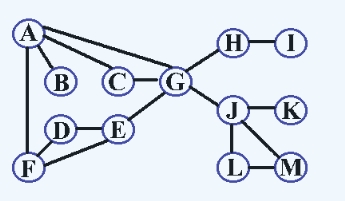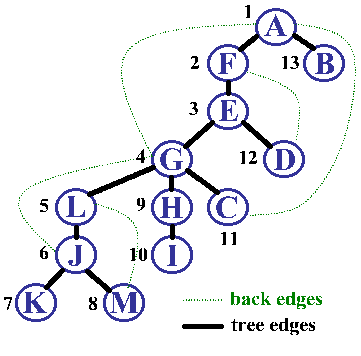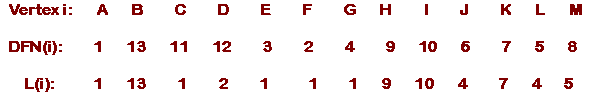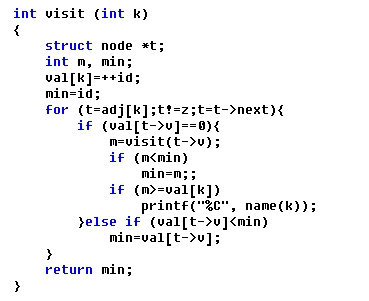Biconnected Graph

 [Basic Concepts] Articulation point: An Articulation point in a connected graph is a vertex that, if delete, would break the graph into two or more pieces (connected component). Biconnected graph: A graph with no articulation point called biconnected. In other words, a graph is biconnected if and only if any vertex is deleted, the graph remains connected. Biconnected component: A biconnected component of a graph is a maximal biconnected subgraph- a biconnected subgraph that is not properly contained in a larger biconnected subgraph. A graph that is not biconnected can divide into biconnected components, sets of nodes mutually accessible via two distinct paths. The graphs we discuss below are all about loop-free undirected ones.Figure 1. The graph G that is not biconnected

 [Example] Graph G in Figure 1: Articulation points: A, H, G, J Biconnected components: {A, C, G, D, E, F}、{G, J, L, B}、B、H、I、K

 How to find articulation points?Figure 2. Depth-first panning tree of the graph G [Step 1.] Find the depth-first spanning tree T for G [Step 2.] Add back edges in T [Step 3.] Determine DNF(i) and L(i) DNF(i): the visiting sequence of vertices i by depth first search L(i): the least DFN reachable frome i through a path consisting of zero or more tree edges followed by zero or one back edge [Step 4.] Vertex i is an articulation point of G if and only if eather: i is the root of T and has at least two children i is not the root and has a child j for which L(j)>=DFN(i)

 [Example] The DFN(i) and L(i) of Graph G in Figure 1 are:Vertex G is an articulation point because G is not the root and in depth-first spanning tree in Figure 2, L(L)>=DFN(G), that L is one of its children Vertex A is an articulation point because A is the root and in depth-first spanning tree in Figure 2, it has more than one child, B and F Vertex E is not an articulation point because E is not the root and in depth-first spanning tree in Figure 2, L(G)

 [Program]